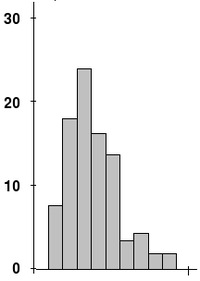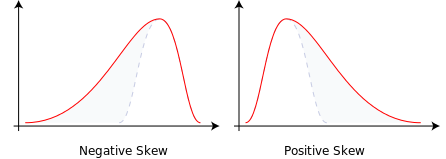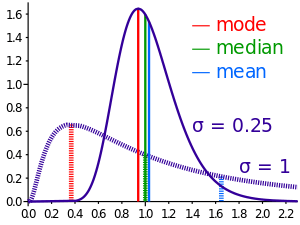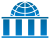# Skewness

For the planarity measure in graph theory, see Graph skewness.Example distribution with non-zero (positive) skewness. These data are from experiments on wheat grass growth.

In probability theory and statistics, skewness is a measure of the asymmetry of the probability distribution of a real-valued random variable about its mean. The skewness value can be positive or negative, or even undefined.

The qualitative interpretation of the skew is complicated. For a unimodal distribution, negative skew indicates that the tail on the left side of the probability density function is longer or fatter than the right side – it does not distinguish these two kinds of shape. Conversely, positive skew indicates that the tail on the right side is longer or fatter than the left side. In cases where one tail is long but the other tail is fat, skewness does not obey a simple rule. For example, a zero value means that the tails on both sides of the mean balance out overall; this is the case for a symmetric distribution, but is also true for an asymmetric distribution where the asymmetries even out, such as one tail being long but thin, and the other being short but fat. Further, in multimodal distributions and discrete distributions, skewness is also difficult to interpret. Importantly, the skewness does not determine the relationship of mean and median. In cases where it is necessary, data might be transformed to have a normal distribution.

## Introduction

Consider the two distributions in the figure just below. Within each graph, the values on the right side of the distribution taper differently from the values on the left side. These tapering sides are called tails, and they provide a visual means to determine which of the two kinds of skewness a distribution has:

1. negative skew: The left tail is longer; the mass of the distribution is concentrated on the right of the figure. The distribution is said to be left-skewed, left-tailed, or skewed to the left.
2. positive skew: The right tail is longer; the mass of the distribution is concentrated on the left of the figure. The distribution is said to be right-skewed, right-tailed, or skewed to the right.Skewness in a data series may sometimes be observed not only graphically but by simple inspection of the values. For instance, consider the numeric sequence (49, 50, 51), whose values are evenly distributed around a central value of 50. We can transform this sequence into a negatively skewed distribution by adding a value far below the mean, e.g. (40, 49, 50, 51). Similarly, we can make the sequence positively skewed by adding a value far above the mean, e.g. (49, 50, 51, 60).

## Relationship of mean and median

The skewness is not directly related to the relationship between the mean and median: a distribution with negative skew can have its mean greater than or less than the median, and likewise for positive skew.

In the older notion of nonparametric skew, defined aswhere µ is the mean, ν is the median, and σ is the standard deviation, the skewness is defined in terms of this relationship: positive/right nonparametric skew means the mean is greater than (to the right of) the median, while negative/left nonparametric skew means the mean is less than (to the left of) the median. However, the modern definition of skewness and the traditional nonparametric definition do not in general have the same sign: while they agree for some families of distributions, they differ in general, and conflating them is misleading.

If the distribution is symmetric, then the mean is equal to the median, and the distribution has zero skewness. If, in addition, the distribution is unimodal, then the mean = median = mode. This is the case of a coin toss or the series 1,2,3,4,... Note, however, that the converse is not true in general, i.e. zero skewness does not imply that the mean is equal to the median.

Paul T. von Hippel points out: "Many textbooks, teach a rule of thumb stating that the mean is right of the median under right skew, and left of the median under left skew. This rule fails with surprising frequency. It can fail in multimodal distributions, or in distributions where one tail is long but the other is heavy. Most commonly, though, the rule fails in discrete distributions where the areas to the left and right of the median are not equal. Such distributions not only contradict the textbook relationship between mean, median, and skew, they also contradict the textbook interpretation of the median."

## Definition

### Pearson's moment coefficient of skewness

The skewness of a random variable X is the third standardized moment γ1, defined as:where μ is the mean, σ is the standard deviation, E is the expectation operator, μ3 is the third central moment, and κt are the tth cumulants. It is sometimes referred to as Pearson's moment coefficient of skewness, or simply the moment coefficient of skewness, but should not be confused with Pearson's other skewness statistics (see below). The last equality expresses skewness in terms of the ratio of the third cumulant κ3 to the 1.5th power of the second cumulant κ2. This is analogous to the definition of kurtosis as the fourth cumulant normalized by the square of the second cumulant. The skewness is also sometimes denoted Skew[X].

Skewness can be expressed in terms of the non-central moment E[X3] by expanding the previous formula,### Examples

Skewness can be infinite, as whenwhere the third cumulants are infinite, or as whenwhere the third cumulant is undefined.

### Properties

Starting from a standard cumulant expansion around a normal distribution, one can show that

skewness = 6 (meanmedian)/standard deviation (1 + kurtosis/8) + O(skewness2).

If Y is the sum of n independent and identically distributed random variables, all with the distribution of X, then the third cumulant of Y is n times that of X and the second cumulant of Y is n times that of X, so. This shows that the skewness of the sum is smaller, as it approaches a Gaussian distribution in accordance with the central limit theorem. Note that the assumption that the variables be independent for the above formula is very important because it is possible even for the sum of two Gaussian variables to have a skewed distribution (see this example).

### Sample skewness

For a sample of n values, a natural method of moments estimator of the population skewness iswhereis the sample mean, s is the sample standard deviation, and the numerator m3 is the sample third central moment.

Another common definition of the sample skewness iswhereis the unique symmetric unbiased estimator of the third cumulant andis the symmetric unbiased estimator of the second cumulant (i.e. the variance).

In general, the ratiosandare both biased estimators of the population skewness; their expected values can even have the opposite sign from the true skewness. (For instance, a mixed distribution consisting of very thin Gaussians centred at −99, 0.5, and 2 with weights 0.01, 0.66, and 0.33 has a skewness of about −9.77, but in a sample of 3,has an expected value of about 0.32, since usually all three samples are in the positive-valued part of the distribution, which is skewed the other way.) Nevertheless,andeach have obviously the correct expected value of zero for any symmetric distribution with a finite third moment, including a normal distribution.

Under the assumption that the underlying random variableis normally distributed, it can be shown that. The variance of the skewness of a random sample of size n from a normal distribution isAn approximate alternative is 6/n, but this is inaccurate for small samples.

In normal samples,has the smaller variance of the two estimators, withwhere m2 in the denominator is the (biased) sample second central moment.

The adjusted Fisher–Pearson standardized moment coefficientis the version found in Excel and several statistical packages including Minitab, SAS and SPSS.

## Applications

Skewness has benefits in many areas. Many models assume normal distribution; i.e., data are symmetric about the mean. The normal distribution has a skewness of zero. But in reality, data points may not be perfectly symmetric. So, an understanding of the skewness of the dataset indicates whether deviations from the mean are going to be positive or negative.

D'Agostino's K-squared test is a goodness-of-fit normality test based on sample skewness and sample kurtosis.

## Other measures of skewnessComparison of mean, median and mode of two log-normal distributions with different skewness.

Other measures of skewness have been used, including simpler calculations suggested by Karl Pearson (not to be confused with Pearson's moment coefficient of skewness, see above). These other measures are:

### Pearson's first skewness coefficient (mode skewness)

The Pearson mode skewness, or first skewness coefficient, is defined as

.

### Pearson's second skewness coefficient (median skewness)

The Pearson median skewness, or second skewness coefficient, is defined as

3 (meanmedian)/standard deviation.

The latter is a simple multiple of the nonparametric skew.

### Quantile-based measures

Bowley's measure of skewness (from 1901), also called Yule's coefficient (from 1912) is defined as:.

When writing it as, it is easier to see that the numerator is the average of the upper and lower quartiles (a measure of location) minus the median while the denominator is (Q3-Q1)/2 which (for symmetric distributions) is the MAD measure of dispersion.

Other names for this measure are Galton's measure of skewness, the Yule–Kendall index and the quartile skewness ,

A more general formulation of a skewness function was described by Groeneveld, R. A. and Meeden, G. (1984):where F is the cumulative distribution function. This leads to a corresponding overall measure of skewness defined as the supremum of this over the range 1/2  u < 1. Another measure can be obtained by integrating the numerator and denominator of this expression. The function γ(u) satisfies −1  γ(u)  1 and is well defined without requiring the existence of any moments of the distribution.

Bowley's measure of skewness is γ(u) evaluated at u = 3/4. Kelley's measure of skewness uses u = 0.1.

### Groeneveld & Meeden’s coefficient

Groeneveld & Meeden have suggested, as an alternative measure of skewness,where μ is the mean, ν is the median, |…| is the absolute value, and E() is the expectation operator. This is closely related in form to Pearson's second skewness coefficient.

### L-moments

Use of L-moments in place of moments provides a measure of skewness known as the L-skewness.

### Distance skewness

A value of skewness equal to zero does not imply that the probability distribution is symmetric. Thus there is a need for another measure of asymmetry that has this property: such a measure was introduced in 2000. It is called distance skewness and denoted by dSkew. If X is a random variable taking values in the d-dimensional Euclidean space, X has finite expectation, X' is an independent identically distributed copy of X, anddenotes the norm in the Euclidean space, then a simple measure of asymmetry isand dSkew(X) := 0 for X = 0 (with probability 1). Distance skewness is always between 0 and 1, equals 0 if and only if X is diagonally symmetric (X and −X have the same probability distribution) and equals 1 if and only if X is a nonzero constant with probability one. Thus there is a simple consistent statistical test of diagonal symmetry based on the sample distance skewness:### Medcouple

The medcouple is a scale-invariant robust measure of skewness, with a breakdown point of 25%. It is the median of the values of the kernel functiontaken over all couplessuch that, whereis the median of the sample.Wikimedia Commons has media related to Skewness (statistics).
1. Susan Dean, Barbara Illowsky "Descriptive Statistics: Skewness and the Mean, Median, and Mode", Connexions website
2. "1.3.5.11. Measures of Skewness and Kurtosis". NIST. Retrieved 18 March 2012.
3. von Hippel, Paul T. (2005). "Mean, Median, and Skew: Correcting a Textbook Rule". Journal of Statistics Education. 13 (2).
4. "Measures of Shape: Skewness and Kurtosis", 2008–2016 by Stan Brown, Oak Road Systems
5. Pearson's moment coefficient of skewness, FXSolver.com
6. Joanes, D. N.; Gill, C. A. (1998). "Comparing measures of sample skewness and kurtosis". Journal of the Royal Statistical Society (Series D): The Statistician. 47 (1): 183–189. doi:10.1111/1467-9884.00122.
7. Duncan Cramer (1997) Fundamental Statistics for Social Research. Routledge. ISBN 9780415172042 (p 85)
8. Kendall, M.G.; Stuart, A. (1969) The Advanced Theory of Statistics, Volume 1: Distribution Theory, 3rd Edition, Griffin. ISBN 0-85264-141-9 (Ex 12.9)
9. Doane DP, Seward LE (2011) J Stat Educ 19 (2)
10. http://www.stat.upd.edu.ph/s114%20cnotes%20fcapistrano/Chapter%2010.pdf
11. Doane, David P., and Lori E. Seward. "Measuring Skewness: A Forgotten Statistic?" Journal of Statistics Education 19.2 (2011): 1-18.
12. Bowley, A. L. (1901). Elements of Statistics, P.S. King & Son, Laondon. Or in a later edition: BOWLEY, AL. "Elements of Statistics, 4th Edn (New York, Charles Scribner)."(1920).
13. Kenney JF and Keeping ES (1962) Mathematics of Statistics, Pt. 1, 3rd ed., Van Nostrand, (page 102).
14. Yule, George Udny. An introduction to the theory of statistics. C. Griffin, limited, 1912.
15. Groeneveld, Richard A. "An influence function approach to describing the skewness of a distribution." The American Statistician 45.2 (1991): 97-102.
16. Johnson et al (1994) p 3, p 40
17. Wilks DS (1995) Statistical Methods in the Atmospheric Sciences, p 27. Academic Press. ISBN 0-12-751965-3
18. Groeneveld, R.A.; Meeden, G. (1984). "Measuring Skewness and Kurtosis". The Statistician. 33 (4): 391–399. doi:10.2307/2987742. JSTOR 2987742.
19. MacGillivray (1992)
20. Hinkley DV (1975) "On power transformations to symmetry", Biometrika, 62, 101–111
21. Hosking, J.R.M. (1992). "Moments or L moments? An example comparing two measures of distributional shape". The American Statistician. 46 (3): 186–189. doi:10.2307/2685210. JSTOR 2685210.
22. Szekely, G.J. (2000). "Pre-limit and post-limit theorems for statistics", In: Statistics for the 21st Century (eds. C. R. Rao and G. J. Szekely), Dekker, New York, pp. 411–422.
23. Szekely, G. J. and Mori, T. F. (2001) "A characteristic measure of asymmetry and its application for testing diagonal symmetry", Communications in Statistics – Theory and Methods 30/8&9, 1633–1639.
• Premaratne, G., Bera, A. K. (2001).

Adjusting the Tests for Skewness and Kurtosis for Distributional Misspecifications. Working Paper Number 01-0116, University of Illinois. Forthcoming in Comm in Statistics, Simulation and Computation.2016 1-15

• Premaratne, G., Bera, A. K. (2000). Modeling Asymmetry and Excess Kurtosis in Stock Return Data. Office of Research Working Paper Number 00-0123, University of Illinois
• Johnson, NL, Kotz, S, Balakrishnan N (1994) Continuous Univariate Distributions, Vol 1, 2nd Edition Wiley ISBN 0-471-58495-9
• MacGillivray, HL (1992). "Shape properties of the g- and h- and Johnson families". Comm. Statistics — Theory and Methods. 21: 1244–1250.
• Skewness Measures for the Weibull DistributionWikiversity has learning materials about Skewness